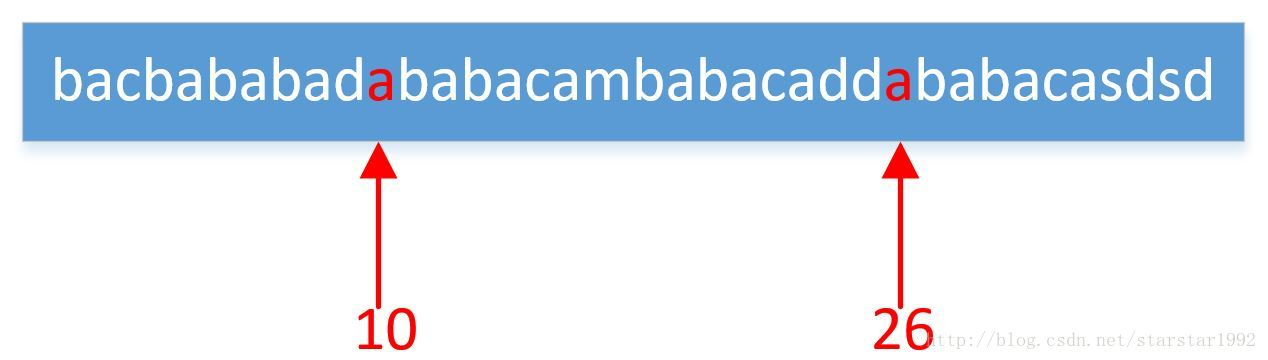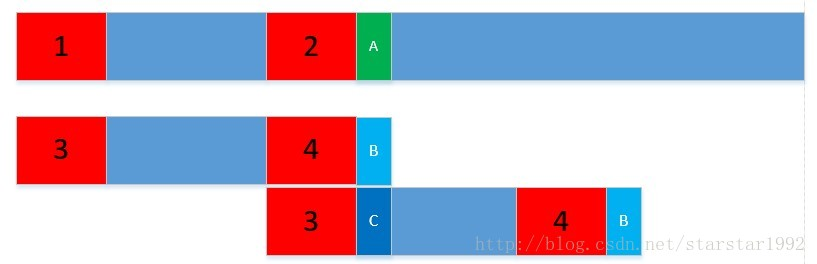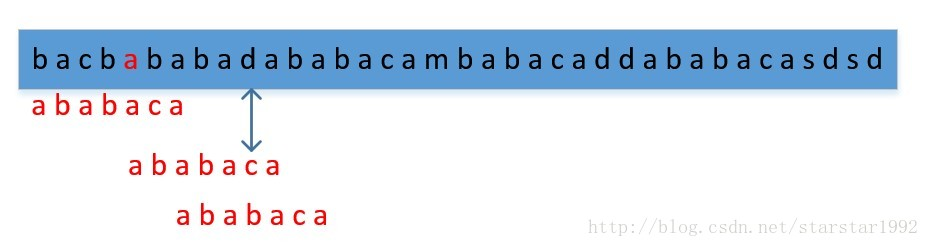# KMP算法浅显理解

### 说明：转载

KMP算法看懂了觉得特别简单，思路很简单，看不懂之前，查各种资料，看的稀里糊涂，即使网上最简单的解释，依然看的稀里糊涂。

KMP算法求解什么类型问题

char *ptr = "ababaca";

str有两处包含ptr### 问题类型很简单，下面直接介绍算法 算法说明

KMP算法：可以实现复杂度为O(m+n)

### next数组的含义就是一个固定字符串的最长前缀和最长后缀相同的长度。

cbcbc，最长前缀和最长后缀相同是cbc。
abcbc，最长前缀和最长后缀相同是不存在的。

**注意最长前缀：是说以第一个字符开始，但是不包含最后一个字符。

a，ab，aba，abab，ababa，ababac，ababaca的相同的最长前缀和最长后缀的长度。由于a，ab，aba，abab，ababa，ababac，ababaca的相同的最长前缀和最长后缀是“”，“”，“a”，“ab”，“aba”，“”，“a”,所以next数组的值是[-1,-1,0,1,2,-1,0]，这里-1表示不存在，0表示存在长度为1，2表示存在长度为3。这是为了和代码相对应。### 代码解析：


void cal_next(char *str, int *next, int len)
{
next = -1;//next初始化为-1，-1表示不存在相同的最大前缀和最大后缀
int k = -1;//k初始化为-1
for (int q = 1; q <= len-1; q++)
{
while (k > -1 && str[k + 1] != str[q])//如果下一个不同，那么k就变成next[k]，注意next[k]是小于k的，无论k取任何值。
{
k = next[k];//往前回溯
}
if (str[k + 1] == str[q])//如果相同，k++
{
k = k + 1;
}
next[q] = k;//这个是把算的k的值（就是相同的最大前缀和最大后缀长）赋给next[q]
}
}

KMP

int KMP(char *str, int slen, char *ptr, int plen)
{
int *next = new int[plen];
cal_next(ptr, next, plen);//计算next数组
int k = -1;
for (int i = 0; i < slen; i++)
{
while (k >-1&& ptr[k + 1] != str[i])//ptr和str不匹配，且k>-1（表示ptr和str有部分匹配）
k = next[k];//往前回溯
if (ptr[k + 1] == str[i])
k = k + 1;
if (k == plen-1)//说明k移动到ptr的最末端
{
//cout << "在位置" << i-plen+1<< endl;
//k = -1;//重新初始化，寻找下一个
//i = i - plen + 1;//i定位到该位置，外层for循环i++可以继续找下一个（这里默认存在两个匹配字符串可以部分重叠），感谢评论中同学指出错误。
return i-plen+1;//返回相应的位置
}
}
return -1;
}

### KMP算法代码：



#include "iostream"
#include "cstring"
using namespace std;

void cal_next(char *str,int *next,int len)
{
next=-1;
int k=-1;
for(int i=1;i<=len-1;i++)
{
while(k>-1&&str[i]!=str[k+1])
k=next[k];
if(str[k+1]==str[i]);
k++;
next[i]=k;
}
}
int KMP(char *str,int slen,char *ptr,int plen)
{
int *next=new int [plen];
cal_next(ptr,next,plen);
int k=-1;
for(int i=0;i<=slen-1;i++)
{
while(k>-1&&ptr[k+1]!=str[i])
k=next[k];
if(ptr[k+1]==str[i])
k++;
if(k==plen-1)
{
return i-plen+1;
}
}
return -1;
}
int main()
{
char str,ptr;
cin>>str;
cin>>ptr;
int a=KMP(str,strlen(str),ptr,strlen(ptr));
if(a==-1)
{
cout<<"找不到"<<endl;
}
else
{
cout<<a<<endl;
}
return 0;
}

### 无关笔记：

class Calor
{
public:
Calor(double a,double b)
{
weight=a;
worth=b;
}
void Getin(const Calor &a);
void Getout(const Calor &a);
void Objshow();
static void init();
static void Allshow() ;//静态函数不能含有cv限定符 const voletile
static void remind();
private:
double weight;
double worth;
static double Aweight;//static不可以重复使用
static double Aworth;
};

double Calor::Aweight=1000;
double Calor::Aworth=1000;//再类里的静态变量，定义如左边

void Calor::Allshow()
{
//    int a=5;静态函数里面使用变量和静态变量都没关系
//    static int b=2;
//    int c=a+b;
cout<<"*******************"<<endl;
cout<<"所剩余货物重量为:"<<Aweight<<"千克"<<endl;
cout<<"所剩余货物价值为:"<<Aworth<<"元"<<endl;
cout<<"*******************"<<endl;
}

class A
{
public:
A(int x,int y):a(x)//静态变量不能成员初始化法
{//const函数不可以与静态函数一起使用，只能是成员函数，而且函数内部只能实现输入输出
cout<<a<<' '<<b;
}
private:
int a;
static int b;
};
int A::b=1;   

02-0724万+11-22216
08-25
04-14811
04-22
04-27
03-1048万+